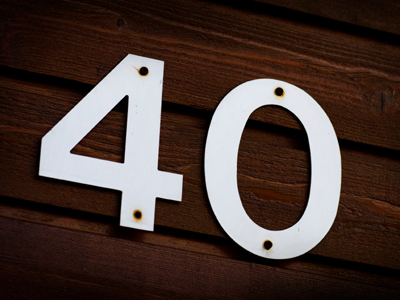10 x 4 = 40.

# 4 Times Table

Learning the times tables is a vital tool when multiplying numbers. By the end of Year 2 children are often fluent in their times tables up to 10. When they enter into KS2 in Year 3 they will also be taught some higher times tables in their Maths and Numeracy lessons. By the end of KS2 they will be familiar with all of the times tables from one to twelve. This quiz will test their knowledge of the 4 times table.

Learning the 4 times table is all about multiplying numbers by 4. One way of learning your times tables is to practise them over and over again until you know them by heart, this will help you in later life when you will have to multiply numbers more often than you think!

Test how well you know your 4 times table by playing the following quiz.

1.
3 x 4 =
6
12
15
18
There are 12 people on every jury
2.
9 x 4 =
30
32
36
40
3.
5 x 4 =
10
16
20
30
4.
8 x 4 =
28
30
32
38
8 x 4 can be written as either 8 + 8 + 8 + 8 or 4 + 4 + 4 + 4 + 4 + 4 + 4 + 4
5.
12 x 4 =
16
40
46
48
6.
7 x 4 =
21
25
28
30
7.
6 x 4 =
12
24
26
28
To times a number by 6, first times it by 10, next half it and finally add the first number once more. So, 4 x 10 = 40, 40 ÷ 2 = 20 and 20 + 4 = 24
8.
2 x 4 =
4
6
8
12
To multiply a number by 2 just double it
9.
11 x 4 =
4
40
42
44
To multiply a number by 11 just times it by 10 and then add it again, so 4 x 10 = 40, 40 + 4 = 44
10.
4 x 4 =
8
16
32
36